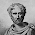## Sunday, 3 June 2012

### Scala: View Bound and Context Bounds

View Bound

A view bound is Scala's mechanism to enable the use of some type A as if it were some type T (Real type). The typical syntax is this:

def func[A <% T](a: A) = a.methodFromT

To affect this, A must an implicit conversion to T so calling method on A calls implicit conversion A to T and then call real methodFromT.
Imagine that for some enteties in the system we want to add side effect: be able to print their state to Console, here is simple example implementation via VIew Bound construction:
1. Implementing class Echo that is going to do our business - present a value: A as a String:

class Echo[A](value: A) {
def echo() = println(value.toString)
}

2. Declaring implicit conversion from Into to Echo

implicit def int2echo (i:Int) : Echo[Int] = new Echo(i)

3. Declaring side effect method that can print anything that has an implicit conversion to Echo

def f[A <% Echo[A]](a: A) = a.echo()

4. Using this method:

f(3)
// prints 3 to Console

As U can find we just extended functionality of class Int to class Echo. Source code of Int isn't touched.

### Variants:

As you will find View Boud is just a syntax sugar for currying with implicit parameter. Despite View Bound is quite old one solution a lot of libraries aren't using it but currying with implicit conversion.

def f1[A <% Echo[A]](a: A) = println(a.echo())
def f2[A](a: A)(implicit convert: A => Echo[A]) = println(convert(a).echo())

### When:

View bound is used mostly to implement the Pimp My Library pattern. In the situations when U want to add methods (behavior) to an existing class (might U don't have access to source code), in situations where you want to return the original type somehow. If you do not need to return that type in any way, then you do not need a view bound.

## Context Bounds

Context Bounds actually generalize View Bounds. It's covers almost the same problems and using the same solution: implicit conversion. It was introduced in Scala 2.8.0 Version and is mostly is used to implement Haskell's type classes, of course with Scala's specific way.
While a view bound can be used with any classes, even simple types (for example, A <% String), a context bound requires a parameterized type, such asEcho[A]above, but never String or Int. It is used to summon some class that can work with our type A.
Here is example:

1. Class Echo has a major changes comparing to previous example. It doesn't wraps a value A but declares a service method that accepts value of type A.

class Echo[A]() {
def echo(a: A) = a.toString
}

2. We are declaring variable of type Echo[Int] that can be injected as implicit parameter to method on demand.

implicit val intEcho = new Echo[Int]()

3. While a A requires availability of paramererized type Echo[A], implicitly method summons (via implicit declaration) instance of Echo[A] and then we can call it methods. Method g declares that type A has an has an associated Echo

def g[A: Echo](a: A) = println(implicitly[Echo[A]].echo(a))

4. Putting it together
g(3) // Prints 3 to Console

### Variants:

Context Bound as a View Bound is a syntax sugar for currying with implicit parameter. Here are desugar example:

def g1[A: Echo](a: A) = println(implicitly[Echo[A]].echo(a))
def g2[A](a: A)(implicit instance: Echo[A]) = println(instance.echo(a))

### When:

Main target is implementation of type classes. Idea of this pattern is implementation of alternative to OO inheritance by making functionality available through a sort of implicit adapter pattern.
One of the popular examples in the past is Ordering:

def func[A : Ordering](a: A, b: A) = if (implicitly[Ordering[A]].lt(a, b)) a else b

and old one example with Numeric:
def func[A : Numeric](a: A, b: A) = implicitly[Numeric[A]].plus(a, b)

Another example is linked to Scala's magic changes with array creation of type T. An Array initialization on a parameterized type requires a ClassManifest to be available, for arcane reasons related to type erasure and the non-erasure nature of arrays.
Runtime information is necessary to create the right representation of Array[T]. One needs to provide this information by passing a ClassManifest[T] into the method as an implicit parameter:

def tabulate[T](len: Int, f: Int => T)(implicit m: ClassManifest[T]) = {
val xs = new Array[T](len)
for (i <- 0 until len) xs(i) = f(i)
xs
}
As a shorthand form, a context bound can be used on the type parameter T instead, giving:

def tabulate[T: ClassManifest](len: Int, f: Int => T) = {
val xs = new Array[T](len)
for (i <- 0 until len) xs(i) = f(i)
xs
}

1.2.